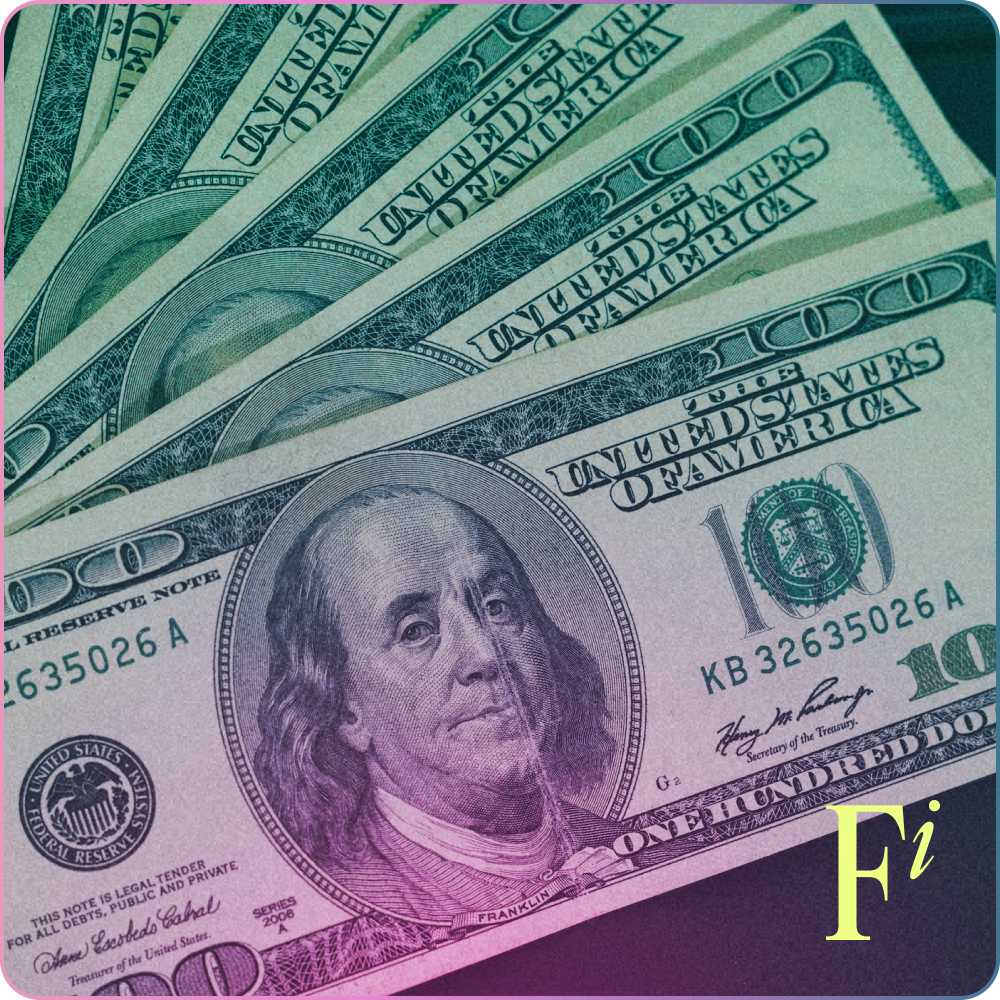<- Back

# Yield

Yield refers to the annual net profit made on your original investment on a particular security, usually in the form of interest or dividend payments.## What is yield?

In other words, your return on an investment over a period of time. Yield is typically measured on an annual basis and is expressed as a percentage, unlike a return which is expressed as a dollar value. The percentage is determined by measuring the cost of the investment against the current market value. There’s lots of ways to measure yield so we know it can feel a bit complicated at times. Just remember the overall concept in the first instance! You don’t need to be an expert in these terms to invest.

KEY TAKEAWAYS

• Yield refers to the annual net profit made on your original investment on a particular security
• It's typically accumulated in the form of interest or dividend payments (or both)!
• It's measured on a yearly basis and expressed as a percentage
• You can calculate the yield of stocks, bonds and dividends## Shares vs bond yields

### Shares

Shares and bonds are most commonly referred to in yields. Share yields are usually given as a percentage of the current share price of a company. That means that an investor who sees the value of their shares increase will see greater return on their investment if dividend levels remain constant.

### Bond yields

Bond yields can be given as either a percentage of the bond price when it is issued, a percentage of the current price of the bond, or an estimate of what the bond’s yield will be if it is held to maturity.

### How is stock yield calculated?

Yield is calculated differently, depending on whether you’re calculating the yield of a stock or bond. For stocks, yield is calculated as a security's price increase plus dividends, divided by the purchase prices.

### How is bond yield calculated?

For bonds, yield can be analyzed as either cost yield or current yield. The cost yield measures the returns as a percentage of the original price of the bond, while current yield is measured in relation to the current price.

### Formula for dividend yield‍

Dividend yield estimates the returns only through the forms of dividends of a stock investment. Since it’s dependent on how well the stock is performing, they can look deceivingly large if the stock price plummets in value and low if the stock price increases.

Dividend yield = annual dividends per share / price per share

Because dividends are paid quarterly, many investors simply take the quarterly dividend, and multiply it by four in order to work out the annual dividend yield calculation.

### Stock yield

The yield on stock investments can be calculated based on either the overall price increase or by dividends paid out by the company. So let’s say you purchase a £80 per share and after a year they sell it for £100, that would be your overall price yield. But that same stock may pay out quarterly dividends, (for example, £2 per share). In that case, the yield would be calculated in the share prices return, plus any dividends paid throughout the year. Then it would be divided by the price you bought the stock for.

We are committed to educating and empowering women to take control of their finances and to live life on their own terms.

CONTACT